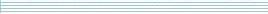| home      | people     | research     | publications    | seminars    | events     | contactCommunication Networks   | Systems Biology   | Hybrid Systems    | Machine Learning   | Dynamics & Interaction### Schools Mathematics Grand Challenge

Week four's Puzzles

Problem 10:

The tenth problem was:

Three women go into a bread shop one after another. The first woman buys half of the total number of loaves in the shop and an extra half loaf (So if there were 21 loaves in the shop, she buys 10.5 + 0.5 = 11 loaves). The second woman buys half of the loaves left in the shop and an extra half loaf. The third woman then goes in and buys half of the remaining loaves and an extra half loaf. When the third woman leaves the shop, there are no loaves left. How many loaves were in the shop to start with?

The solution was:

The answer is 7. Let x,y,z be the number of loaves bought by the first, second and third woman respectively. The total number of loaves in the shop is then x+y+z. The first woman takes half of the total number of loaves in the shop plus an extra half loaf:

x = (x+y+z)/2 + 1/2

At this point, there are (x+y+z)/2 - 1/2 loaves left in the shop.

Now the second woman comes in and takes half of what is left plus an extra half loaf:

y = (x+y+z)/4 + 1/4

At this point, there are (x+y+z)/4 - 3/4 loaves left in the shop.

Finally, the third woman comes in and takes half of what is left plus an extra half loaf:

z = (x+y+z)/8 + 1/8

After she leaves there no bread left. This means that

(x+y+z)/4 - 3/4 - (x+y+z)/8 - 1/8 = 0

or

(x+y+z)/8 - 7/8 = 0

or (x+y+z) = 7.

Thus the first woman takes 4 loaves, the second woman 2, and the third woman 1.

Problem 11:

The eleventh problem was:

John, Mary, Thomas and Sheila all have mobile phones with the same company, so all four of them have different numbers. One day, while quite bored, they decide to play the following game. First, each of them subtracts the numbers of the other three people from their own number, then multiplies the three resulting differences together, and finally divides 1 by the result.

So, for instance, if the numbers of the four friends were J (for John's number), M (for Mary's number), T (for Thomas' number), S (for Sheila's number), then John would calculate

1 divided by the product (J - M)x(J - T)x(J - S),

while Mary would calculate

1 divided by the product (M - J)x(M - T)x(M - S).

If John, Mary, Thomas and Sheila add up the four answers obtained in this way, what will the sum be?

The solution was:

The sum is zero (0). To see this, let us introduce the shorthand notation

a := (J-M)
b := (J-T)
c := (J-S)

Note that we can write all other differences in terms of a,b, and c, as follows:

(M-J) = -a
(M-T) = b-a
(M-S) = c-a

(T-J) = -b
(T-M) = a-b
(T-S) = c-b

(S-J) = -c
(S-M) = a-c
(S-T) = b-c

The sum can then be written as

sum = 1/(abc) + 1/((a-b)(a-c)(b-c))*[-(b-c)/a + (a-c)/b + (a-b)/c]

The term in square brackets [] can be rewritten as

[...] = 1/(abc)*{-(b-c)bc + (a-c)ac - (a-b)ab}

The term in curly brackets {} can be rewritten as

{...} = -(a-b)(a-c)(b-c)

Collecting terms we get

sum = 1/(abc) + 1/((a-b)(a-c)(b-c))*[{-(a-b)(a-c)(b-c)}/(abc)],

or simply

sum = 1/(abc) - 1/(abc)

In other words, we find that sum = 0.

Problem 12:

The twelveth problem was:

The number 14 is the smallest whole number with the following two properties:

1. It is bigger than 10;
2. It is smaller than the sum of the squares of its digits - (1 x 1) + (4 x 4) = 1 + 16 = 17 > 14.

What is the largest whole number satisfying these two properties?

The solution was:

The answer is 99.

It is easy to see that there is no 4-digit number with these properties, because with 4 digits the sum of squares can never exceed 4*9*9 = 324, while by definition all 4-digit numbers are greater than or equal to 1000, which is strictly greater than 324. A similar argument applies for all numbers with more digits.

What about 3-digit numbers? Can we find a number with 3 digits, d1, d2, and d3, such that

d1*100 + d2*10 + d3*1 < d1*d1 + d2*d2 + d3*d3?

Or equivalently, can we find d1, d2, d3, such that

d1*(100 - d1) + d2*(10-d2) + d3*(1-d3) < 0?

The answer is no. Remember d1 > 0 (when d1=0 we have a 2-digit number!), we have that

d1*(100 - d1) ≥ 99
d2*(10 - d2) ≥ 0
d3*(1 - d3) ≥ -72

When we add it all up, we find that

d1*(100 - d1) + d2*(10-d2) + d3*(1-d3) >= 27

Since 27 is strictly greater than 0, we conclude that there exists no three-digit number with the above mentioned properties. Now consider the case of two-digit numbers. We try 99 and find that it works (because 99 < 81+81). Since 99 is the largest 2-digit number and since we know that there is no number with 3 or more digits that satisfies the desired properties, 99 must be the large whole number that does.